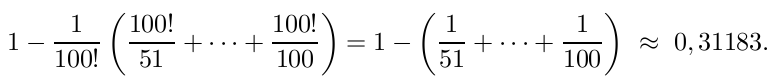The probability for a random permutation of n = 100,     that all cycles have length at most 50, is ≈ 31 %.

Sketch of proof.
For t > 50, the number of permutations p
with a cycle of length t, is 100!/t (see below).

Thus, the probability for p to have no cycle of length > 50 is:The number of permutations with a cycle of length t>50 is 100!/t.

Proof: A permutation of 100 has at most one cycle of length t.

There are binom(100,t) possibilities for choosing the numbers for the cycle.
There are (t-1)! possibilities to order the numbers inside the cycle.
There are (100-t)! permutations of the remaining numbers.

binom(100,t) × (t-1)! × (100-t)! = 100!/t.